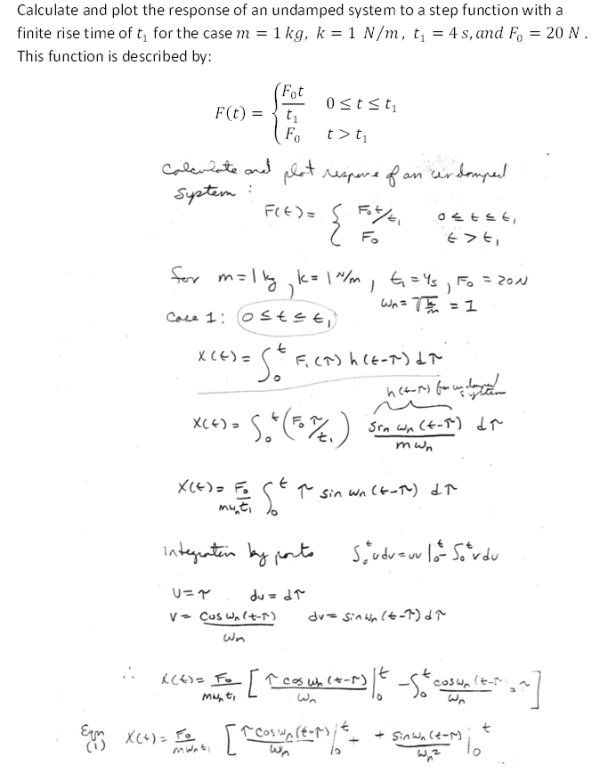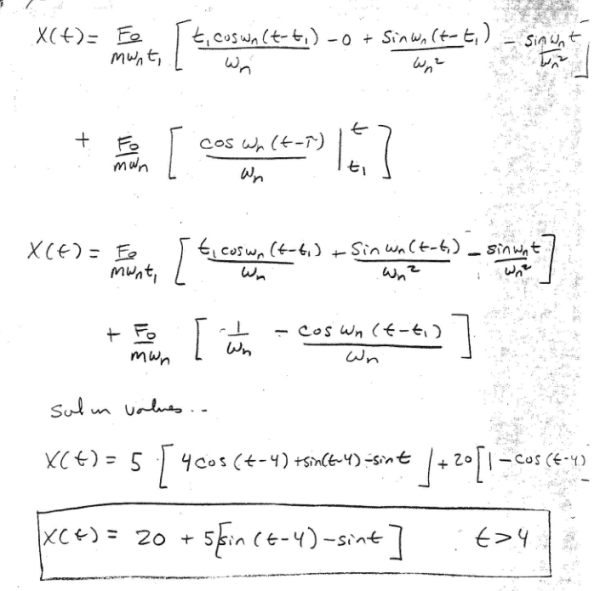Calculate and plot the response of an undamped system to a step function with a finite rise time of t1 for the case m=1 kg, k=1 N/m, t1=4s, and F0=20 N. This function is described by: F(t)=F0t/t1 and F0Calculate and plot the response of an undamped system to a step function with a finite rise time of t1 for the case m=1 kg, k=1 N/m, t1=4s, and F0=20 N. This function is described by: F(t)=F0t/t1 and F0

Vibrations Page 1 vibrations vibrations vibrations vibrations vibrations vibrations vibrations Vibrations Page 2